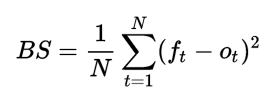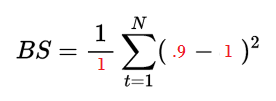# Brier Score: Definition, Examples

Statistics Definitions > Brier Score

## What is a Brier Score?

A brier score is a way to verify the accuracy of a probability forecast. A probability forecast refers to a specific event, such as there is a 25% probability of it raining in the next 24 hours. The score can only be used for binary outcomes, where there are only two possible events, like “it rained” or “it didn’t rain.” It could also be used for categorical outcomes as long as they can be structured as binary outcomes (i.e. “true” or “false”).

• The best possible Brier score is 0, for total accuracy.
• The lowest possible score is 1, which mean the forecast was wholly inaccurate.

Smaller scores (closer to zero) indicate better forecasts. Scores in the middle (e.g. 0.44, 0.69) can be hard to interpret as “good” or “bad”, so these are sometimes converted to Brier skill scores.

## Calculating the Brier Score

The most common formula is the mean squared error:Where:

• N = the number of items you’re calculating a Brier score for.
• ft is the forecast probability (i.e. 25% chance),
• ot is the outcome (1 if it happened, 0 if it didn’t).
• Σ is the summation symbol. It just means to “add up” all of the values.

If you have one value in your sample, there’s no need to add anything. The formula just becomes:

Brier score = (Actual result – Forecast Probability)

But if you have several samples, you’ll want to calculate the squared differences (ft – ot)2 first, then add those up, and then divide by N.

Sample question:
The forecast for rain yesterday was 90%. It did rain (At 3 p.m.). What is the Brier score for the forecast?

Step 1: Insert the figures into the formula. The forecast was 90% (.9) and it rained, so ot is 1:Step 2: Solve:
(.9 – 1)2 = .01

## Brier Skill Scores

Brier scores can tell you how accurate a forecast was, but don’t tell you how accurate they were compared with anything else. For example, although the score might be high, a forecast using historical data might actually be better. A solution is to use the Brier skill score. While a Brier score answers the question “how large was the error in the forecast?”, the Brier skill score answers the question:

“What is the relative skill of the probabilistic forecast over that of climatology, in terms of predicting whether or not an event occurred?.”

The formula is:

BSS = 1 – BS/BSref

Where:

• BS = Brier score
• BSref = BS for the reference forecast.

A “reference forecast” is usually a long-term forecast or similar statistic. Caution should be used when interpreting the score with small samples, as this could make the denominator very small. For rare events, you’ll need a larger sample size.

A Brier skill score has a range of -∞ to 1.

• Negative values mean that the forecast is less accurate than a standard forecast.
• 0 = no skill compared to the reference forecast.
• 1 = perfect skill compared to the reference forecast.

Reference:
World Climate Research Programme.

CITE THIS AS:
Stephanie Glen. "Brier Score: Definition, Examples" From StatisticsHowTo.com: Elementary Statistics for the rest of us! https://www.statisticshowto.com/brier-score/